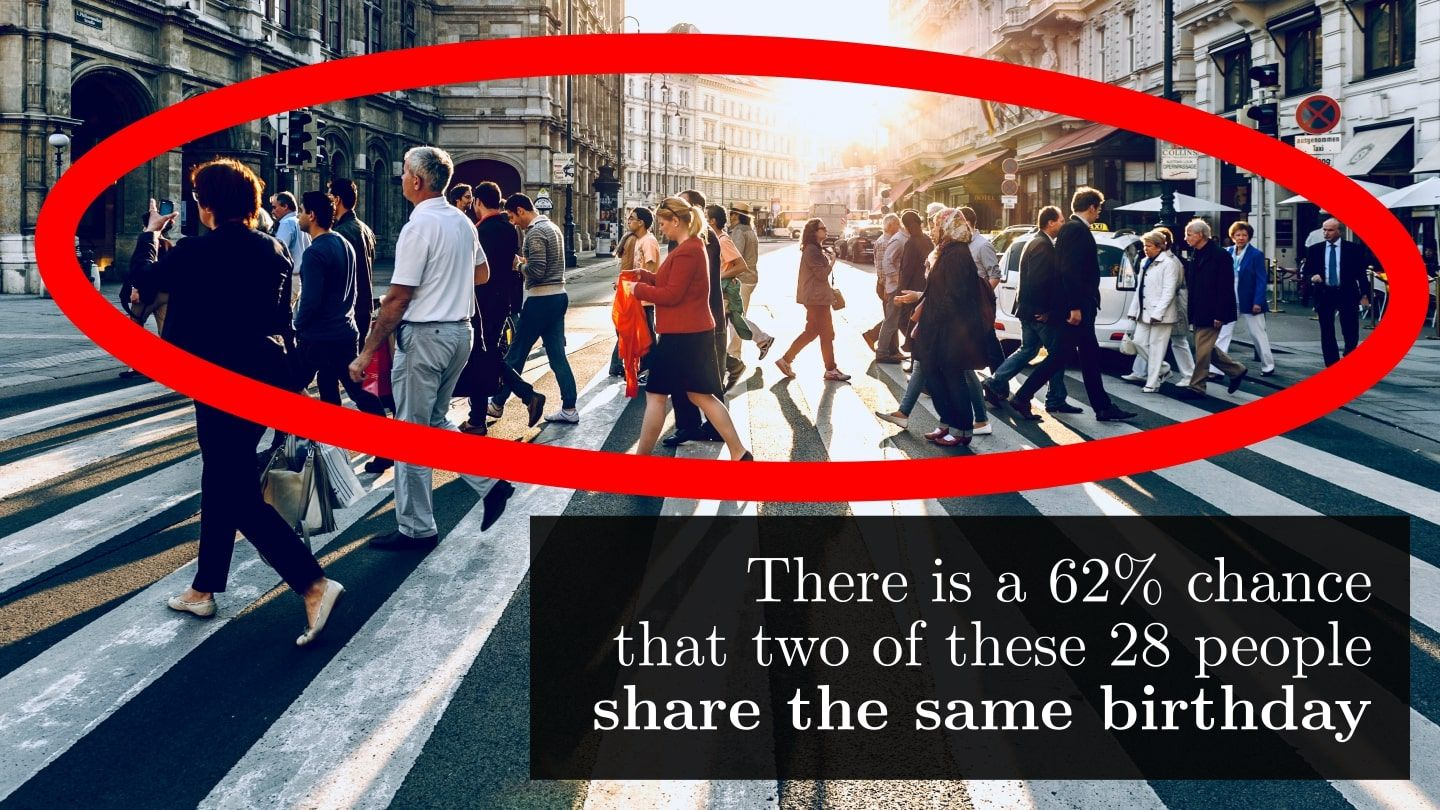There is a $62\%$ chance that two of these $28$ people share the same birthday.

This is quite shocking, as most of us would guess the chance to be less than $10\%$

Here is why our intuition is wrong.

The usual thinking is if there are $28$ people and $365$ days in a year, then chances should be roughly $27/365 \approx 7.4\%$, and if we have $366$ people, then it is guaranteed that two of them share birthdays.

However, this is not how probability works.

First, it is much easier to talk about the probability of having no shared birthdays.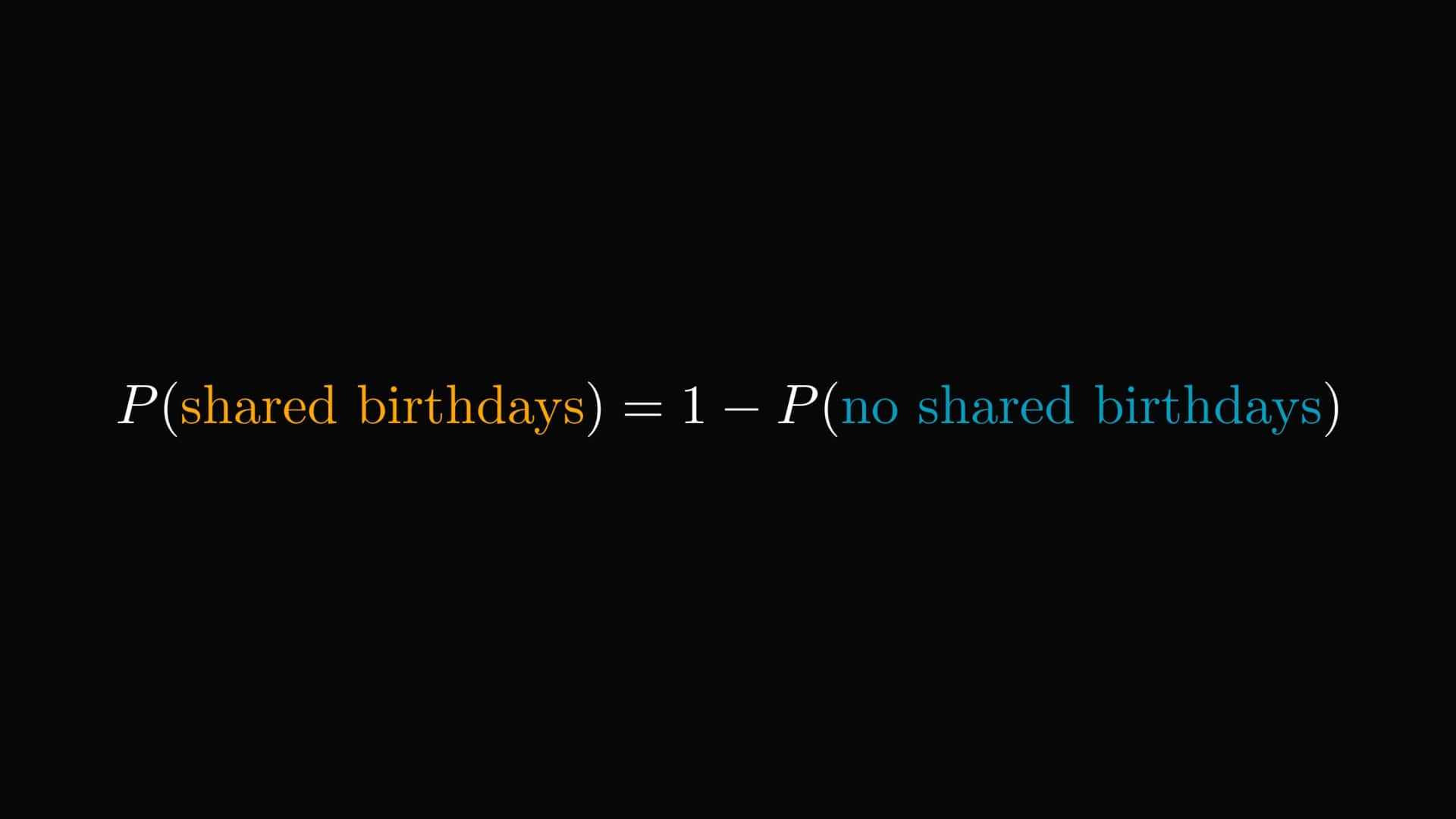This is a common trick, often making the calculations much more manageable.

Let's simplify the problem even more.

Given two people, what is the probability of sharing the same birthday?

By encoding the birthday with an integer between $1$ and $365$, we can count the number of configurations.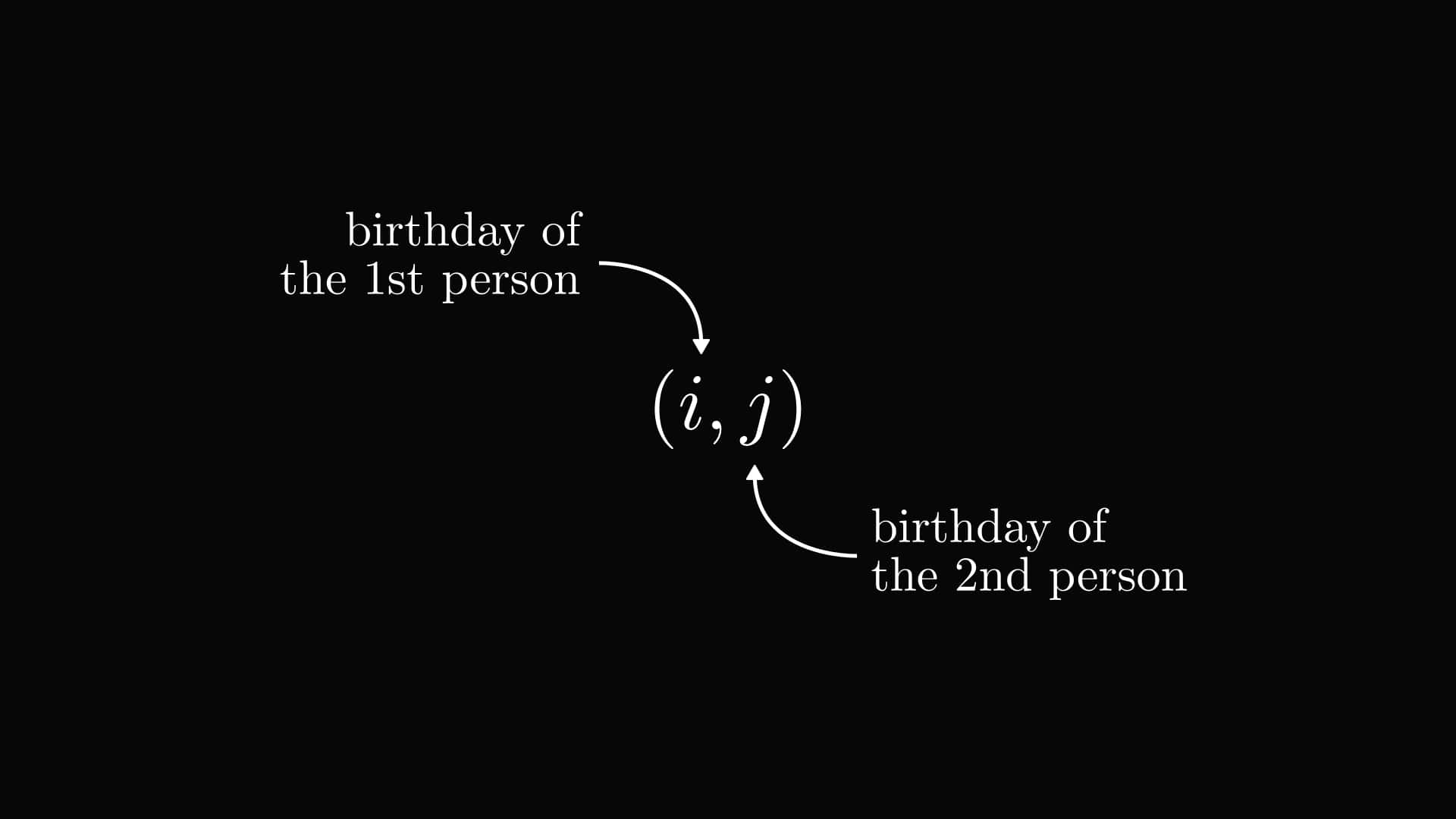How many days can we pick as the 1st element of the tuple? $365$.

How many days can we pick as the 2nd element to avoid the birthday collision? $364$.

In total, there are $365 \cdot 364$ ways.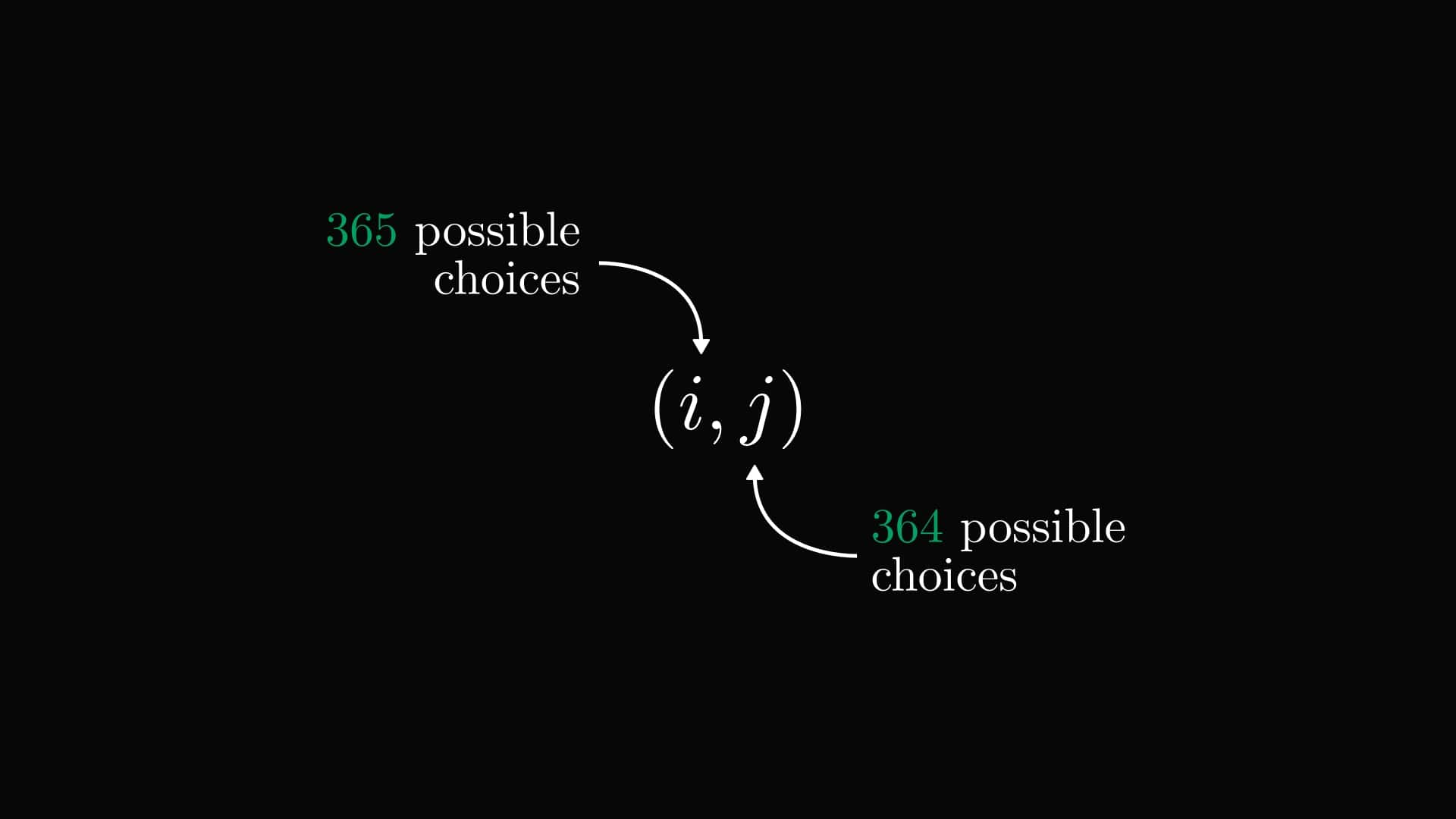To count the number of total configurations, we disregard the potential birthday collision. Thus, there are $365 \cdot 365$ possibilities.

The probability is the ratio of the number of desired outcomes and all possible outcomes.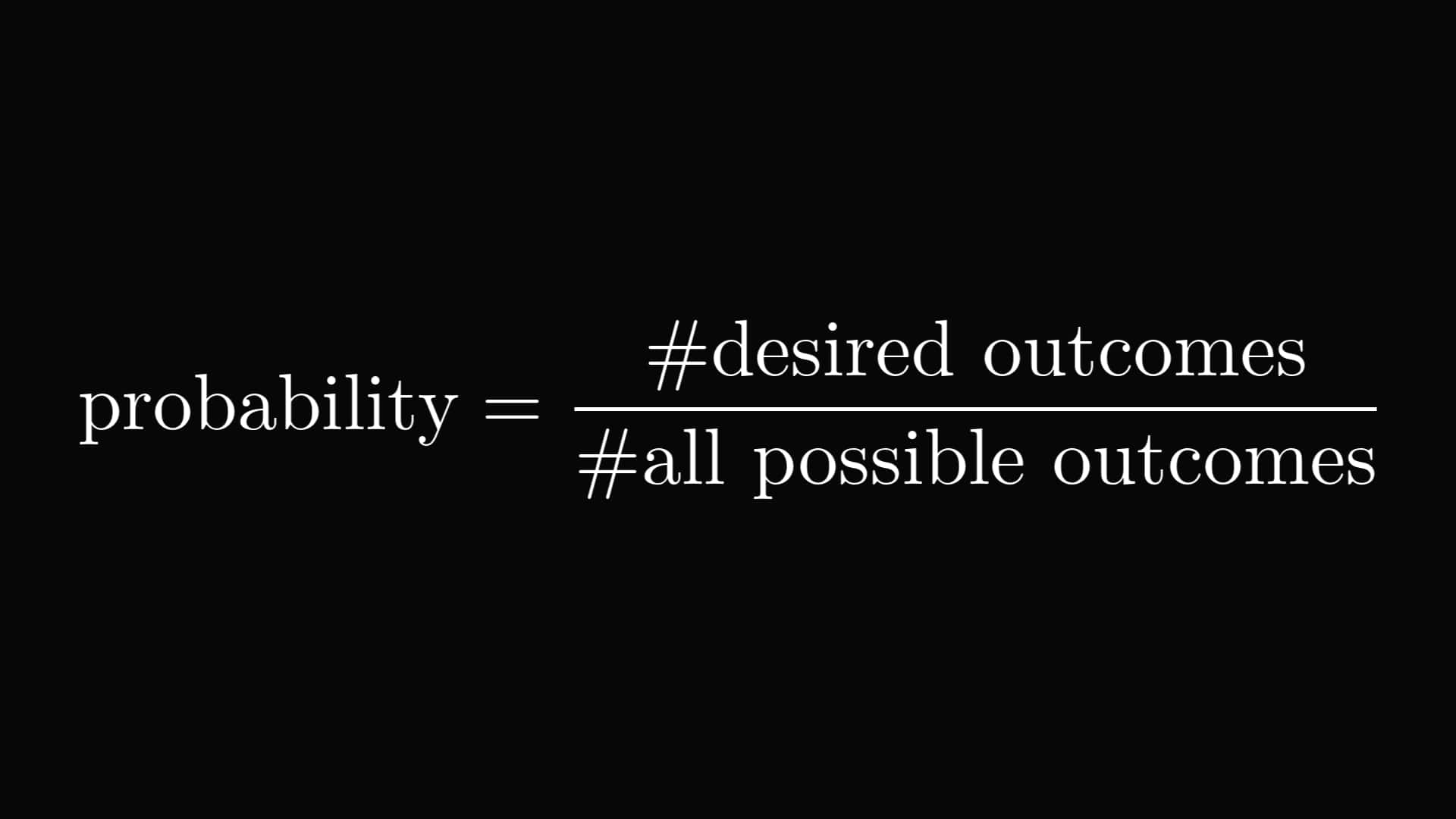In this case, the result is the following. Summing up, the probability of two people sharing the same birthdays is less than one percent.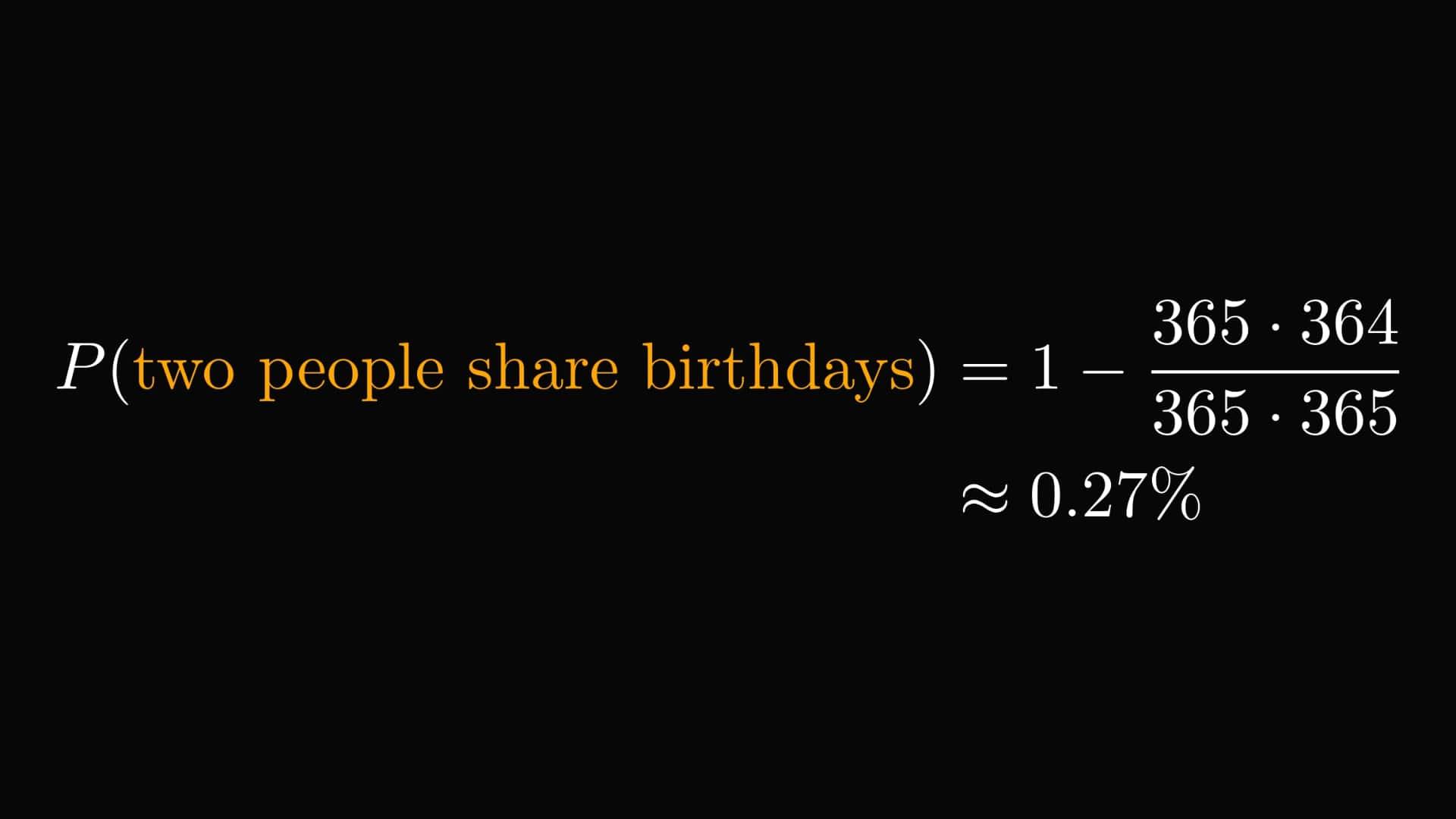What about the general case? Following the same logic, we can solve the general problem.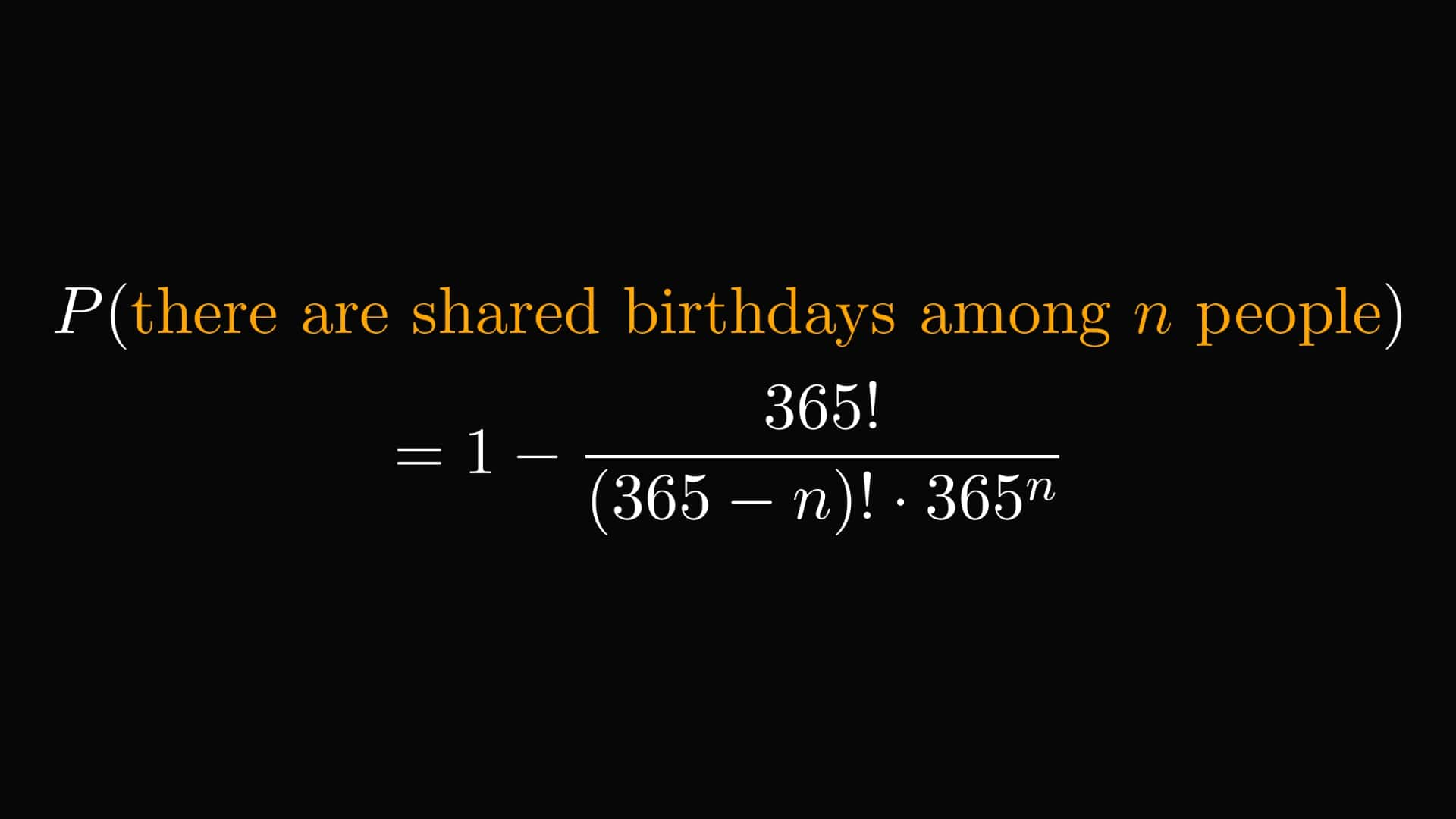At $n = 23$ people, the probability reaches $50\%$.

At $n = 41$, the probability is $90\%$.

Quite surprising, isn't it?

If you are curious, this is what the probability distribution looks like.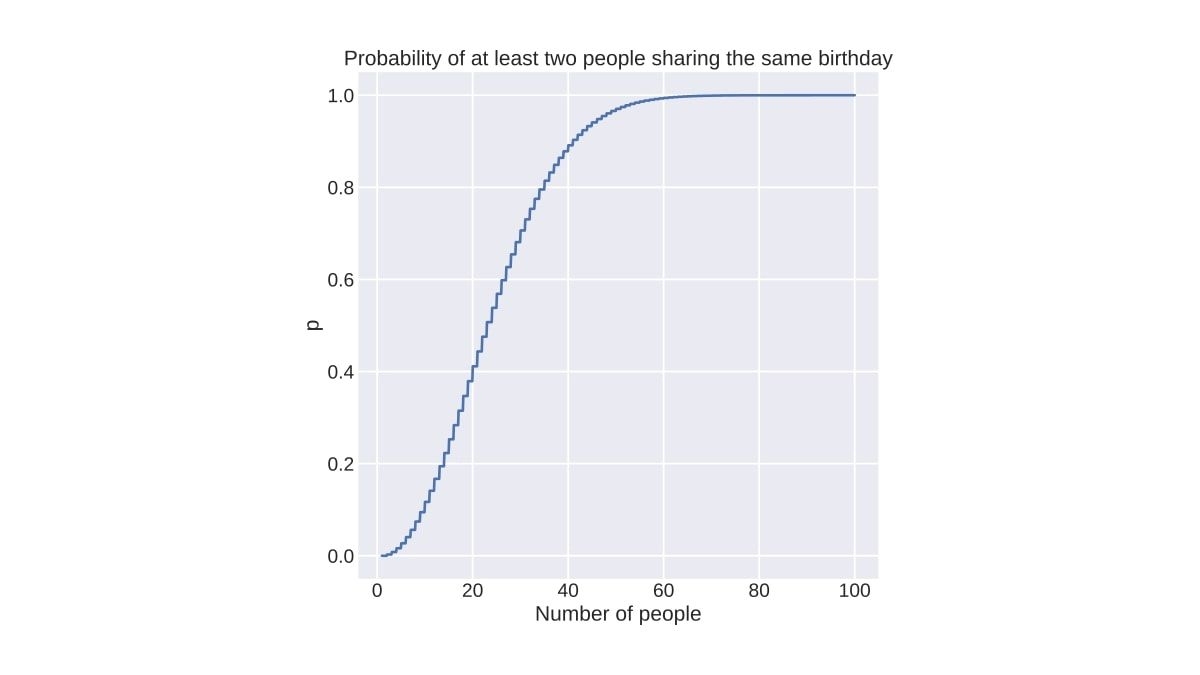What can we learn from the birthday problem?

That our intuition about probability often fails hard. Most estimate the chances of having a shared birthday among $25$ people very low. The actual probability is more than $50\%$.